# Impedance Matching and Smith Chart Impedance

### Abstract

Tutorial on RF impedance matching using the Smith chart. Examples are shown plotting reflection coefficients, impedances and admittances. A sample matching network of the MAX2472 is designed at 900MHz using graphical methods.

Tried and true, the Smith chart is still the basic tool for determining transmission-line impedances.

When dealing with the practical implementation of RF applications, there are always some nightmarish tasks. One is the need to match the different impedances of the interconnected blocks. Typically these include the antenna to the low-noise amplifier (LNA), power-amplifier output (RFOUT) to the antenna, and LNA/VCO output to mixer inputs. The matching task is required for a proper transfer of signal and energy from a "source" to a "load."

At high radio frequencies, the spurious elements (like wire inductances, interlayer capacitances, and conductor resistances) have a significant yet unpredictable impact on the matching network. Above a few tens of megahertz, theoretical calculations and simulations are often insufficient. In-situ RF lab measurements, along with tuning work, have to be considered for determining the proper final values. The computational values are required to set up the type of structure and target component values.

There are many ways to do impedance matching, including:

• Computer simulations: Complex but simple to use, as such simulators are dedicated to differing design functions and not to impedance matching. Designers have to be familiar with the multiple data inputs that need to be entered and the correct formats. They also need the expertise to find the useful data among the tons of results coming out. In addition, circuit-simulation software is not pre-installed on computers, unless they are dedicated to such an application.
• Manual computations: Tedious due to the length ("kilometric") of the equations and the complex nature of the numbers to be manipulated.
• Instinct: This can be acquired only after one has devoted many years to the RF industry. In short, this is for the super-specialist.

The primary objectives of this article are to review the Smith chart's construction and background, and to summarize the practical ways it is used. Topics addressed include practical illustrations of parameters, such as finding matching network component values. Of course, matching for maximum power transfer is not the only thing we can do with Smith charts. They can also help the designer with such tasks as optimizing for the best noise figures, ensuring quality factor impact, and assessing stability analysis.### A Quick Primer

Before introducing the Smith chart utilities, it would be prudent to present a short refresher on wave propagation phenomenon for IC wiring under RF conditions (above 100MHz). This can be valid for contingencies such as RS-485 lines, between a PA and an antenna, between an LNA and a downconverter/mixer, and so forth.

It is well known that, to get the maximum power transfer from a source to a load, the source impedance must equal the complex conjugate of the load impedance, or:

• RS + jXS = RL - jXLFor this condition, the energy transferred from the source to the load is maximized. In addition, for efficient power transfer, this condition is required to avoid the reflection of energy from the load back to the source. This is particularly true for high-frequency environments like video lines and RF and microwave networks.

### What It Is

A Smith chart is a circular plot with a lot of interlaced circles on it. When used correctly, matching impedances, with apparent complicated structures, can be made without any computation. The only effort required is the reading and following of values along the circles.

The Smith chart is a polar plot of the complex reflection coefficient (also called gamma and symbolized by Γ). Or, it is defined mathematically as the 1-port scattering parameter s or s11.

A Smith chart is developed by examining the load where the impedance must be matched. Instead of considering its impedance directly, you express its reflection coefficient ΓL, which is used to characterize a load (such as admittance, gain, and transconductance). The ΓL is more useful when dealing with RF frequencies.

We know the reflection coefficient is defined as the ratio between the reflected voltage wave and the incident voltage wave:The amount of reflected signal from the load is dependent on the degree of mismatch between the source impedance and the load impedance. Its expression has been defined as follows: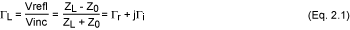Because the impedances are complex numbers, the reflection coefficient will be a complex number as well.

In order to reduce the number of unknown parameters, it is useful to freeze the ones that appear often and are common in the application. Here Z0 (the characteristic impedance) is often a constant and a real industry normalized value, such as 50Ω, 75Ω, 100Ω, and 600Ω. We can then define a normalized load impedance by: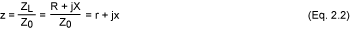With this simplification, we can rewrite the reflection coefficient formula as: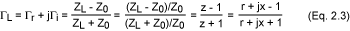Here we can see the direct relationship between the load impedance and its reflection coefficient. Unfortunately, the complex nature of the relation is not useful practically, so we can use the Smith chart as a type of graphical representation of the above equation.

To build the chart, the equation must be rewritten to extract standard geometrical figures (like circles or stray lines).

First, equation 2.3 is reversed to give: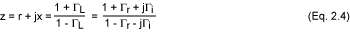andBy setting the real parts and the imaginary parts of equation 2.5 equal, we obtain two independent, new relationships: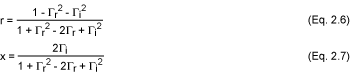Equation 2.6 is then manipulated by developing equations 2.8 through 2.13 into the final equation, 2.14. This equation is a relationship in the form of a parametric equation (x - a)² + (y - b)² = R² in the complex plane (Γr, Γi) of a circle centered at the coordinates [r/(r + 1), 0] and having a radius of 1/(1 + r).See Figure 4a for further details.When developing the Smith chart, there are certain precautions that should be noted. These are among the most important:

• All the circles have one same, unique intersecting point at the coordinate (1, 0).
• The zero Ω circle where there is no resistance (r = 0) is the largest one.
• The infinite resistor circle is reduced to one point at (1, 0).
• There should be no negative resistance. If one (or more) should occur, we will be faced with the possibility of oscillatory conditions.
• Another resistance value can be chosen by simply selecting another circle corresponding to the new value.

### Back to the Drawing Board

Moving on, we use equations 2.15 through 2.18 to further develop equation 2.7 into another parametric equation. This results in equation 2.19.Again, 2.19 is a parametric equation of the type (x - a)² + (y - b)² = R² in the complex plane (Γr, Γi) of a circle centered at the coordinates (1, 1/x) and having a radius of 1/x.

See Figure 4b for further details.### Get the Picture?

To complete our Smith chart, we superimpose the two circles' families. It can then be seen that all of the circles of one family will intersect all of the circles of the other family. Knowing the impedance, in the form of r + jx, the corresponding reflection coefficient can be determined. It is only necessary to find the intersection point of the two circles corresponding to the values r and x.

### It's Reciprocating Too

The reverse operation is also possible. Knowing the reflection coefficient, find the two circles intersecting at that point and read the corresponding values r and × on the circles. The procedure for this is as follows:

• Determine the impedance as a spot on the Smith chart.
• Find the reflection coefficient (Γ) for the impedance.
• Having the characteristic impedance and Γ, find the impedance.
• Convert the impedance to admittance.
• Find the equivalent impedance.
• Find the component values for the wanted reflection coefficient (in particular the elements of a matching network, see Figure 7).

### To Extrapolate

Because the Smith chart resolution technique is basically a graphical method, the precision of the solutions depends directly on the graph definitions. Here is an example that can be represented by the Smith chart for RF applications:

Example: Consider the characteristic impedance of a 50Ω termination and the following impedances:

 Z1 = 100 + j50Ω Z2 = 75 - j100Ω Z3 = j200Ω Z4 = 150Ω Z5 = ∞ (an open circuit) Z6 = 0 (a short circuit) Z7 = 50Ω Z8 = 184 - j900Ω

Then, normalize and plot (see Figure 5). The points are plotted as follows:

 z1 = 2 + j z2 = 1.5 - j2 z3 = j4 z4 = 3 z5 = 8 z6 = 0 z7 = 1 z8 = 3.68 - j18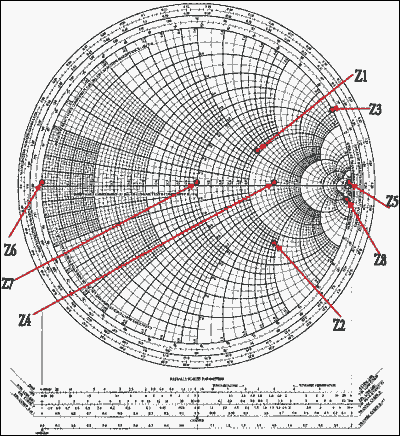For Larger Image (PDF)

It is now possible to directly extract the reflection coefficient Γ on the Smith chart of Figure 5. Once the impedance point is plotted (the intersection point of a constant resistance circle and of a constant reactance circle), simply read the rectangular coordinates projection on the horizontal and vertical axis. This will give Γr, the real part of the reflection coefficient, and Γi, the imaginary part of the reflection coefficient (see Figure 6).

It is also possible to take the eight cases presented in the example and extract their corresponding Γ directly from the Smith chart of Figure 6. The numbers are:

 Γ1 = 0.4 + 0.2j Γ2 = 0.51 - 0.4j Γ3 = 0.875 + 0.48j Γ4 = 0.5 Γ5 = 1 Γ6 = -1 Γ7 = 0 Γ8 = 0.96 - 0.1jThe Smith chart is built by considering impedance (resistor and reactance). Once the Smith chart is built, it can be used to analyze these parameters in both the series and parallel worlds. Adding elements in a series is straightforward. New elements can be added and their effects determined by simply moving along the circle to their respective values. However, summing elements in parallel is another matter. This requires considering additional parameters. Often it is easier to work with parallel elements in the admittance world.

We know that, by definition, Y = 1/Z and Z = 1/Y. The admittance has been expressed in mhos or Ω-1, though now is expressed as siemens, or S. And, as Z is complex, Y must also be complex.

Therefore, Y = G + jB (2.20), where G is called "conductance" and B the "susceptance" of the element. It's important to exercise caution, though. By following the logical assumption, we can conclude that G = 1/R and B = 1/X. This, however, is not the case. If this assumption is used, the results will be incorrect.

When working with admittance, the first thing that we must do is normalize y = Y/Y0. This results in y = g + jb. So, what happens to the reflection coefficient? By working through the following: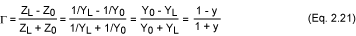It turns out that the expression for G is the opposite, in sign, of z, and Γ(y) = -Γ(z).

If we know z, we can invert the signs of Γ and find a point situated at the same distance from (0, 0), but in the opposite direction. This same result can be obtained by rotating an angle 180° around the center point (see Figure 7).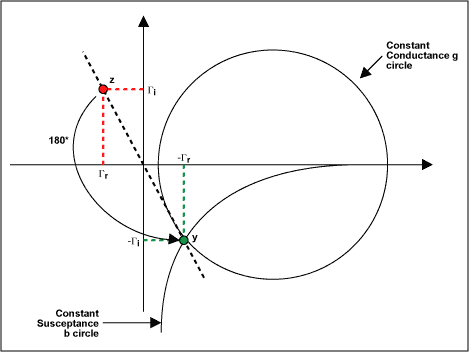Of course, while Z and 1/Y do represent the same component, the new point appears as a different impedance (the new value has a different point in the Smith chart and a different reflection value, and so forth). This occurs because the plot is an impedance plot. But the new point is, in fact, an admittance. Therefore, the value read on the chart has to be read as siemens.

Although this method is sufficient for making conversions, it doesn't work for determining circuit resolution when dealing with elements in parallel.

In the previous discussion, we saw that every point on the impedance Smith chart can be converted into its admittance counterpart by taking a 180° rotation around the origin of the Γ complex plane. Thus, an admittance Smith chart can be obtained by rotating the whole impedance Smith chart by 180°. This is extremely convenient, as it eliminates the necessity of building another chart. The intersecting point of all the circles (constant conductances and constant susceptances) is at the point (-1, 0) automatically. With that plot, adding elements in parallel also becomes easier. Mathematically, the construction of the admittance Smith chart is created by: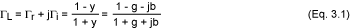then, reversing the equation:Next, by setting the real and the imaginary parts of equation 3.3 equal, we obtain two new, independent relationships:By developing equation 3.4, we get the following:which again is a parametric equation of the type (x - a)² + (y - b)² = R² (equation 3.12) in the complex plane (Γr, Γi) of a circle with its coordinates centered at [-g/(g + 1), 0] and having a radius of 1/(1 + g).

Furthermore, by developing equation 3.5, we show that: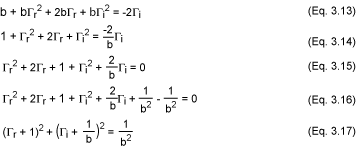which is again a parametric equation of the type (x - a)² + (y - b)² = R² (equation 3.17).

### Equivalent Impedance Resolution

When solving problems where elements in series and in parallel are mixed together, we can use the same Smith chart and rotate it around any point where conversions from z to y or y to z exist.

Let's consider the network of Figure 8 (the elements are normalized with Z0 = 50Ω). The series reactance (x) is positive for inductance and negative for capacitance. The susceptance (b) is positive for capacitance and negative for inductance.The circuit needs to be simplified (see Figure 9). Starting at the right side, where there is a resistor and an inductor with a value of 1, we plot a series point where the r circle = 1 and the l circle = 1. This becomes point A. As the next element is an element in shunt (parallel), we switch to the admittance Smith chart (by rotating the whole plane 180°). To do this, however, we need to convert the previous point into admittance. This becomes A'. We then rotate the plane by 180°. We are now in the admittance mode. The shunt element can be added by going along the conductance circle by a distance corresponding to 0.3. This must be done in a counterclockwise direction (negative value) and gives point B. Then we have another series element. We again switch back to the impedance Smith chart.Before doing this, it is again necessary to reconvert the previous point into impedance (it was an admittance). After the conversion, we can determine B'. Using the previously established routine, the chart is again rotated 180° to get back to the impedance mode. The series element is added by following along the resistance circle by a distance corresponding to 1.4 and marking point C. This needs to be done counterclockwise (negative value). For the next element, the same operation is performed (conversion into admittance and plane rotation). Then move the prescribed distance (1.1), in a clockwise direction (because the value is positive), along the constant conductance circle. We mark this as D. Finally, we reconvert back to impedance mode and add the last element (the series inductor). We then determine the required value, z, located at the intersection of resistor circle 0.2 and reactance circle 0.5. Thus, z is determined to be 0.2 + j0.5. If the system characteristic impedance is 50Ω, then Z = 10 + j25Ω (see Figure 10).

### Matching Impedances by Steps

Another function of the Smith chart is the ability to determine impedance matching. This is the reverse operation of finding the equivalent impedance of a given network. Here, the impedances are fixed at the two access ends (often the source and the load), as shown in Figure 11. The objective is to design a network to insert between them so that proper impedance matching occurs.At first glance, it appears that it is no more difficult than finding equivalent impedance. But the problem is that an infinite number of matching network component combinations can exist that create similar results. And other inputs may need to be considered as well (such as filter type structure, quality factor, and limited choice of components).

The approach chosen to accomplish this calls for adding series and shunt elements on the Smith chart until the desired impedance is achieved. Graphically, it appears as finding a way to link the points on the Smith chart. Again, the best method to illustrate the approach is to address the requirement as an example.

The objective is to match a source impedance (ZS) to a load (zL) at the working frequency of 60MHz (see Figure 11). The network structure has been fixed as a lowpass, L type (an alternative approach is to view the problem as how to force the load to appear as an impedance of value = ZS, a complex conjugate of ZS). Here is how the solution is found.

The first thing to do is to normalize the different impedance values. If this is not given, choose a value that is in the same range as the load/source values. Assume Z0 to be 50Ω. Thus zS = 0.5 - j0.3, z*S = 0.5 + j0.3, and zL = 2 - j0.5.

Next, position the two points on the chart. Mark A for zL and D for z*S.

Then identify the first element connected to the load (a capacitor in shunt) and convert to admittance. This gives us point A'.

Determine the arc portion where the next point will appear after the connection of the capacitor C. As we don't know the value of C, we don't know where to stop. We do, however, know the direction. A C in shunt means to move in the clockwise direction on the admittance Smith chart until the value is found. This will be point B (an admittance). As the next element is a series element, point B has to be converted to the impedance plane. Point B' can then be obtained. Point B' has to be located on the same resistor circle as D. Graphically, there is only one solution from A' to D, but the intermediate point B (and hence B') will need to be verified by a "test-and-try" setup. After having found points B and B', we can measure the lengths of arc A' through B and arc B' through D. The first gives the normalized susceptance value of C. The second gives the normalized reactance value of L. The arc A' through B measures b = 0.78 and thus B = 0.78 × Y0 = 0.0156S. Because ωC = B, then C = B/ω = B/(2πf) = 0.0156/[2π(60 × 106)] = 41.4pF.

The arc B' through D measures x = 1.2, thus X = 1.2 × Z0 = 60Ω. Because ωL = X, then L = X/ω = X/(2πf) = 60/[2π(60 × 106)] = 159nH.A second example matches the output of the MAX2472 with a 50Ω load impedance (zL) at the working frequency of 900MHz (see Figure 14). This network will use the same configuration shown in the MAX2472 data sheet. The above figure shows the matching network with a shunt inductor and a series capacitor. Here is how the solution is found.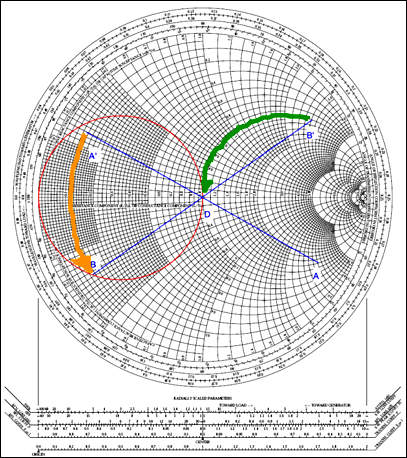The first thing to do is to convert the S22 scattering parameter into its equivalent normalized source impedance. The MAX2472 uses Z0 to be 50Ω. Thus an S22 = 0.81/-29.4° becomes zS = 1.4 - j3.2, zL = 1, and zL* = 1.

Next, position the two points on the chart. Mark A for zS and D for zL*. Because the first element connected to the source is a shunt inductor, convert the source impedance to admittance. This gives us point A'.

Determine the arc portion where the next point will appear after the connection of the inductor LMATCH. As we do not know the value of LMATCH, we do not know where to stop. We do, however, know that after the addition of LMATCH (and a conversion back to impedance), the resulting source impedance should lie on the r = 1 circle. Therefore, the additional series capacitor CMATCH can bring the resulting impedance to z = 1 + j0. By rotating the r = 1 circle 180° about the origin, we plot all the possible admittance values that correspond to the r = 1 circle. The intersection of this reflected circle and the constant conductance circle used with point A' gives us point B (an admittance). The reflection of point B to impedance becomes point B'.

After having found points B and B', we can measure the lengths of arc A' through B and arc B' through D. The first measurement gives the normalized susceptance value of LMATCH. The second gives the normalized reactance value of CMATCH. The arc A' through B measures b = -0.575 and thus B = -0.575 × Y0 = 0.0115S. Because 1/ωL = B, then LMATCH = 1/Bω = 1/(B2πf) = 1/(0.01156 × 2 × π × 900 × 106) = 15.38nH, which rounds to 15nH. The arc B' through D measures × = -2.81, thus X = -2.81 × Z0 = -140.5Ω. Because -1/ωC = X, then CMATCH = -1/Xω = -1/(X2πf) = -1/(-140.5 × 2 × π × 900 × 106) = 1.259pF, which rounds to 1pF. While these calculated values do not take into account parasitic inductances and capacitances of components, they yield values close to the data-sheet specified values of LMATCH = 12nH and CMATCH = 1pF.

### Conclusion

Given today's wealth of software and accessibility of high-speed high-power computers, one may question the need for such a basic and fundamental method for determining circuit fundamentals.

In reality, what makes an engineer a real engineer is not only academic knowledge but also the ability to use resources of all types to solve a problem. It is easy to plug a few numbers into a program and have it spit out the solutions. When the solutions are complex and multifaceted, having a computer to do the grunt work is especially handy. However, knowing underlying theory and principles that have been ported to computer platforms, and where they came from, makes the engineer or designer a more well-rounded and confident professional, and makes the results more reliable.

A similar version of this article appeared in the July 2000 issue of RF Design.